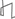Research Abstracts Home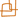CSAIL Digital Archive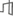Research Activities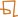CSAIL HomeResearch Abstracts - 2007IntroductionPerception & LearningPhysical, Biological & Social SystemsSystemsTheory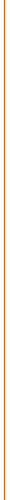### Iteratively Constraining the Marginal Polytope for Approximate Inference and MAP

#### David Sontag & Tommi Jaakkola

##### Background

Markov Random Fields (MRFs) have been useful across a wide spectrum of problems, from computer vision and natural language processing to computational biology. The utility of such models depends critically on fast and accurate inference calculations, typically either finding the most likely setting of all the variables (referred to here as the MAP problem) or evaluating marginal probabilities of specific subsets of the variables. With the exception of low tree-width MRFs, or specific subclasses of models such as restricted planar graphs, solving either of these inference problems requires approximate methods.

We will consider here a subclass of MRFs, those that are naturally expressed in terms of pairwise dependencies (potentials) between the variables. In this context, the challenging nature of inference calculations can be traced back to the difficulty of working with what is known as the marginal polytope M. This is the set of marginal probabilities arising from valid MRFs with the same structure, i.e., marginal probabilities that are realizable.

Finding the MAP assignment for MRFs with pairwise potentials can be cast as an integer linear program over the marginal polytope. This problem is also known as the MAX-CUT problem and has been extensively studied in the mathematical programming literature [1,2,3]. The approximate methods solve the linear program over an easier to handle outer bound on the marginal polytope. For example, the simple linear programming relaxation of the MAP problem corresponds to optimizing over the local marginal polytope characterized by pairwise consistent marginals. Any integral solution to this relaxed problem gives a valid MAP assignment.

The marginal polytope also plays a critical role in approximate (or exact) variational evaluation of marginal probabilities in MRFs (cf. convex duality and the exponential family of distributions ). For example, in the tree-reweighted sum-product (TRW) algorithm of Wainwright et al.  the inference problem is posed as a convex optimization problem over the marginal polytope, then relaxed to an outer bound. The additional difficulty in this context is in representing the entropy function corresponding to any approximate (pseudo) marginal probabilities.

##### Our Approach

The main contribution of our work is to show how to achieve tighter outer bounds on the marginal polytope in an efficient manner using a cutting-plane style algorithm, iterating between solving a relaxed problem and adding additional constraints. While extensively used for solving integer linear programs, such methods have yet to be demonstrated in the context of evaluating marginal probabilities. One key intuition for why this type of algorithm may be successful is that the marginal polytope only needs to be well-specified near the optimum of the objective, and that for real-world problems that have structure, only a small number of constraints may be necessary to sufficiently constrain the marginal polytope near the optimum. The approach can also be used as an anytime algorithm, allowing us to trade-off increased running time for possibly better approximations.

##### Related Work

A number of existing methods for evaluating marginals can be related to approximations of the marginal polytope. Mean field methods, for example, use inner bounds on the marginal polytope by restricting the approximating distributions to lie within specific families of distributions, subsets of the model in question. As a result, one obtains a lower bound on the log-partition function as opposed to an upper bound characteristic of outer bound approximations. The advantage of inner bounds is that for these points within the marginal polytope, e.g., trees or completely factored distributions, the corresponding entropy functions have closed form expressions. The primary disadvantage is the loss of convexity and the accompanying difficulties with locally optimal solutions.

Most message passing algorithms for evaluating marginals, including belief propagation (sum product) and TRW, operate within the local marginal polytope. In TRW, the key contribution involves the entropy function rather than the marginal polytope. The entropy is decomposed into a weighted combination of entropies of tree-structured distributions with the same pairwise marginals. A different type of restriction on the marginal polytope comes from semi-definite constraints (any valid covariance matrix has to be positive semi-definite). Such a restriction on the marginal polytope can be enforced implicitly through a log-determinant approximation to the entropy when evaluating marginals (Wainwright and Jordan ).

Previous work most related to ours is by Barahona et al.  in the context of finding the MAP assignment in Ising models. Their approach iterates between solving the LP and adding in constraints corresponding to violated cycle inequalities [1,2]. Our key observation is that similar ideas can be used to approximately solve any objective function which is defined on the marginal polytope M. In particular, we can use any relaxation to the entropy (e.g. TRW or log-determinant) to find the pseudomarginals.

##### Preliminary Results

A natural question raised by this work is whether optimizing over a tighter relaxation of the marginal polytope would result in improved accuracy for the pseudomarginals, since this proposed algorithm now enables us to efficiently do so. Because we use an approximation to the entropy, it was previously unknown whether tighter outer bounds on the marginal polytope would be helpful. Our first step toward answering this question was to investigate the accuracy of the pseudomarginals when using the exact marginal polytope in combination with either the TRW or log-determinant entropy relaxations.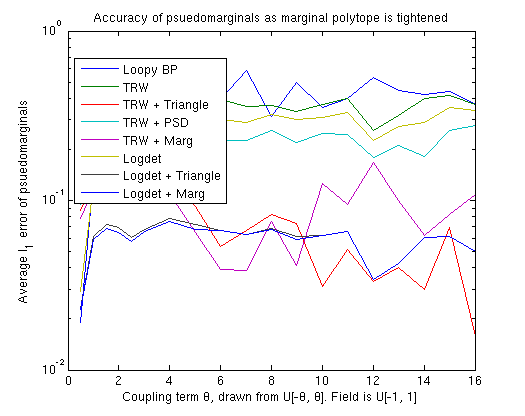The above figure shows the results for Ising models on complete MRFs with 10 variables, where the external field is drawn uniformly in the range [-1,1] and the coupling parameters were drawn uniformly in the range [-θ, θ] (θ is the coupling strength shown in the figure). Note that these highly coupled MRFs are some of the most difficult MRFs to do inference in. For each data point we averaged the results over 10 trials. The Y-axis, given on a log-scale, shows the average l_1 error of the singleton marginals. Note that although the coupling is so large, the external field is also significant, and the actual probabilities are interesting, away from .5 and not all the same (as you would find in a highly coupled model with attractive potentials).

In this difficult setting, loopy belief propagation (with a .5 decay rate) seldom converges. The TRW and log-determinant relaxations result in marginals only slightly better than with loopy belief propagation. However, using the exact marginal polytope significantly improves the accuracy of the approximation. Looking at the polytope relaxation obtained by our algorithm using the cycle inequalities (denoted by "+ Triangle"), we see that it does almost as well as if we had used the exact marginal polytope (denoted by "+ Marg"), and even better in some situations. We conclude that our algorithm has the potential to significantly improve the accuracy of approximate inference for MRFs.

##### Research Support

The authors acknowledge support from the Defense Advanced Research Projects Agency (Transfer Learning program). David Sontag is also supported by a National Science Foundation Graduate Research Fellowship.

##### References:

 F. Barahona and A.R. Mahjoub. On the cut polytope. In Mathematical Programming, vol. 36 pp. 157--173, 1986.

 M. Deza and M. Laurent. Geometry of Cuts and Metrics. In Algorithms and Combinatorics, vol. 15, Springer, 1997.

 S. Chopra and J. Owen. Extended formulations of the A-cut problem. In Mathematical Programming, vol. 73 pp. 7--30, 1996.

 F. Barahona, M. Grotschel, M. Junger, and G. Reinelt. An application of combinatorial optimization to statistical physics and circuit layout design. In Operations Research, vol. 36 pp. 493--513, 1988.

 M. Wainwright, T. Jaakkola, and A. Willsky. A new class of upper bounds on the log partition function. In IEEE Transactions on Information Theory, vol. 51 pp. 2313--2335, 2005.

 M. Wainwright and M. I. Jordan. Log-determinant relaxation for approximate inference in discrete Markov random fields. In IEEE Transactions on Signal Processing, vol. 54 pp. 2099--2109, 2006.

 M. J. Wainwright and M. I. Jordan. Graphical models, exponential families and variational inference. Technical Report 649, UC Berkeley, Dept. of Statistics, 2003.Computer Science and Artificial Intelligence Laboratory (CSAIL) The Stata Center, Building 32 - 32 Vassar Street - Cambridge, MA 02139 - USA tel:+1-617-253-0073 - publications@csail.mit.edu